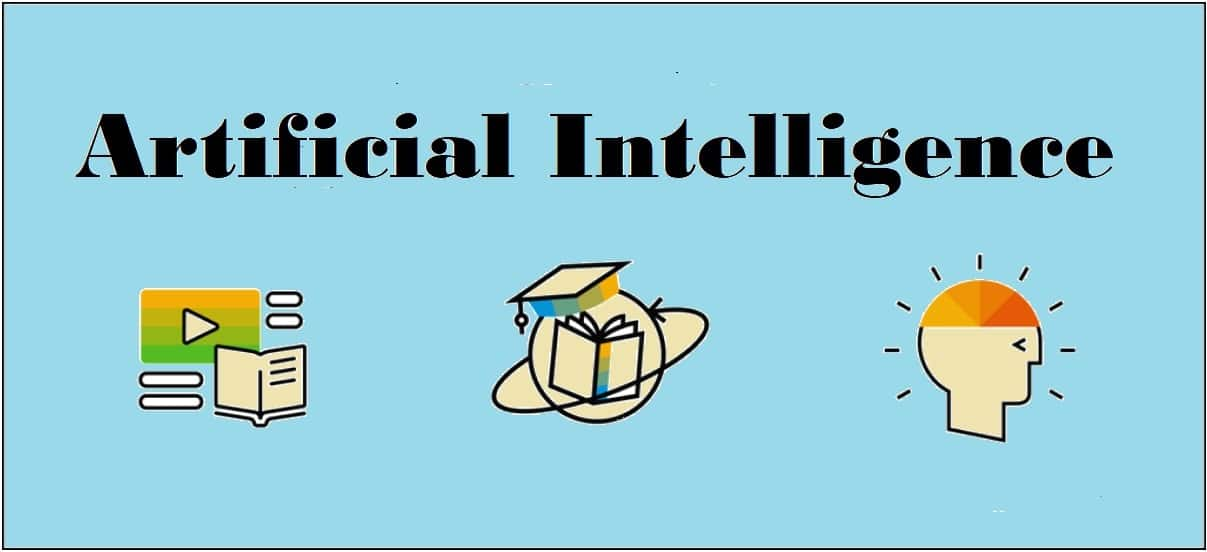## Deep Learning

Deep learning emerged from a decade’s explosive computational growth as a serious contender in the field. Thus, deep learning is a particular kind of machine learning whose algorithms are inspired by the structure and function of human brain.

### Machine Learning vs Deep Learning

Deep learning is the most powerful machine learning technique these days. It is so powerful because they learn the best way to represent the problem while learning how to solve the problem. A comparison of Deep learning and Machine learning is given below −

### Data Dependency

The first point of difference is based upon the performance of DL and ML when the scale of data increases. When the data is large, deep learning algorithms perform very well.

### Machine Dependency

Deep learning algorithms need high-end machines to work perfectly. On the other hand, machine learning algorithms can work on low-end machines too.

### Feature Extraction

Deep learning algorithms can extract high level features and try to learn from the same too. On the other hand, an expert is required to identify most of the features extracted by machine learning.

### Time of Execution

Execution time depends upon the numerous parameters used in an algorithm. Deep learning has more parameters than machine learning algorithms. Hence, the execution time of DL algorithms, specially the training time, is much more than ML algorithms. But the testing time of DL algorithms is less than ML algorithms.

### Approach to Problem Solving

Deep learning solves the problem end-to-end while machine learning uses the traditional way of solving the problem i.e. by breaking down it into parts.

[wpsbx_html_block id=1891]

### Convolutional Neural Network (CNN)

Convolutional neural networks are the same as ordinary neural networks because they are also made up of neurons that have learnable weights and biases. Ordinary neural networks ignore the structure of input data and all the data is converted into 1-D array before feeding it into the network. This process suits the regular data, however if the data contains images, the process may be cumbersome.

CNN solves this problem easily. It takes the 2D structure of the images into account when they process them, which allows them to extract the properties specific to images. In this way, the main goal of CNNs is to go from the raw image data in the input layer to the correct class in the output layer. The only difference between an ordinary NNs and CNNs is in the treatment of input data and in the type of layers.

### Architecture Overview of CNNs

Architecturally, the ordinary neural networks receive an input and transform it through a series of hidden layer. Every layer is connected to the other layer with the help of neurons. The main disadvantage of ordinary neural networks is that they do not scale well to full images.

The architecture of CNNs have neurons arranged in 3 dimensions called width, height and depth. Each neuron in the current layer is connected to a small patch of the output from the previous layer. It is similar to overlaying a 𝑵×𝑵 filter on the input image. It uses M filters to be sure about getting all the details. These M filters are feature extractors which extract features like edges, corners, etc.

### Layers used to construct CNNs

Following layers are used to construct CNNs −

• Input Layer − It takes the raw image data as it is.
• Convolutional Layer − This layer is the core building block of CNNs that does most of the computations. This layer computes the convolutions between the neurons and the various patches in the input.
• Rectified Linear Unit Layer − It applies an activation function to the output of the previous layer. It adds non-linearity to the network so that it can generalize well to any type of function.
• Pooling Layer − Pooling helps us to keep only the important parts as we progress in the network. Pooling layer operates independently on every depth slice of the input and resizes it spatially. It uses the MAX function.
• Fully Connected layer/Output layer − This layer computes the output scores in the last layer. The resulting output is of the size 𝟏×𝟏×𝑳 , where L is the number training dataset classes.

### Installing Useful Python Packages

You can use Keras, which is an high level neural networks API, written in Python and capable of running on top of TensorFlow, CNTK or Theno. It is compatible with Python 2.7-3.6. You can learn more about it from https://keras.io/.

Use the following commands to install keras −

```pip install keras
```

On conda environment, you can use the following command −

```conda install –c conda-forge keras
```

### Building Linear Regressor using ANN

In this section, you will learn how to build a linear regressor using artificial neural networks. You can use KerasRegressor to achieve this. In this example, we are using the Boston house price dataset with 13 numerical for properties in Boston. The Python code for the same is shown here −

Import all the required packages as shown −

```import numpy
import pandas
from keras.models import Sequential
from keras.layers import Dense
from keras.wrappers.scikit_learn import KerasRegressor
from sklearn.model_selection import cross_val_score
from sklearn.model_selection import KFold```

Now, load our dataset which is saved in local directory.

```dataframe = pandas.read_csv("/Usrrs/admin/data.csv", delim_whitespace = True, header = None)
dataset = dataframe.values```

Now, divide the data into input and output variables i.e. X and Y −

```X = dataset[:,0:13]
Y = dataset[:,13]```

Since we use baseline neural networks, define the model −

`def baseline_model():`

Now, create the model as follows −

```model_regressor = Sequential()
model_regressor.add(Dense(13, input_dim = 13, kernel_initializer = 'normal',
activation = 'relu'))

Next, compile the model −

```model_regressor.compile(loss='mean_squared_error', optimizer='adam')
return model_regressor```

Now, fix the random seed for reproducibility as follows −

```seed = 7
numpy.random.seed(seed)```

The Keras wrapper object for use in scikit-learn as a regression estimator is called KerasRegressor. In this section, we shall evaluate this model with standardize data set.

```estimator = KerasRegressor(build_fn = baseline_model, nb_epoch = 100, batch_size = 5, verbose = 0)
kfold = KFold(n_splits = 10, random_state = seed)
baseline_result = cross_val_score(estimator, X, Y, cv = kfold)
print("Baseline: %.2f (%.2f) MSE" % (Baseline_result.mean(),Baseline_result.std()))```

The output of the code shown above would be the estimate of the model’s performance on the problem for unseen data. It will be the mean squared error, including the average and standard deviation across all 10 folds of the cross validation evaluation.

### Image Classifier: An Application of Deep Learning

Convolutional Neural Networks (CNNs) solve an image classification problem, that is to which class the input image belongs to. You can use Keras deep learning library. Note that we are using the training and testing data set of images of cats and dogs from following link https://www.kaggle.com/c/dogs-vs-cats/data.

Import the important keras libraries and packages as shown −

The following package called sequential will initialize the neural networks as sequential network.

`from keras.models import Sequential`

The following package called Conv2D is used to perform the convolution operation, the first step of CNN.

`from keras.layers import Conv2D`

The following package called MaxPoling2D is used to perform the pooling operation, the second step of CNN.

`from keras.layers import MaxPooling2D`

The following package called Flatten is the process of converting all the resultant 2D arrays into a single long continuous linear vector.

`from keras.layers import Flatten`

The following package called Dense is used to perform the full connection of the neural network, the fourth step of CNN.

`from keras.layers import Dense`

Now, create an object of the sequential class.

`S_classifier = Sequential()`

Now, next step is coding the convolution part.

`S_classifier.add(Conv2D(32, (3, 3), input_shape = (64, 64, 3), activation = 'relu'))`

Here relu is the rectifier function.

Now, the next step of CNN is the pooling operation on the resultant feature maps after convolution part.

`S-classifier.add(MaxPooling2D(pool_size = (2, 2)))`

Now, convert all the pooled images into a continuous vector by using flattering −

`S_classifier.add(Flatten())`

Next, create a fully connected layer.

`S_classifier.add(Dense(units = 128, activation = 'relu'))`

Here, 128 is the number of hidden units. It is a common practice to define the number of hidden units as the power of 2.

Now, initialize the output layer as follows −

`S_classifier.add(Dense(units = 1, activation = 'sigmoid'))`

Now, compile the CNN, we have built −

`S_classifier.compile(optimizer = 'adam', loss = 'binary_crossentropy', metrics = ['accuracy'])`

Here optimizer parameter is to choose the stochastic gradient descent algorithm, loss parameter is to choose the loss function and metrics parameter is to choose the performance metric.

Now, perform image augmentations and then fit the images to the neural networks −

```train_datagen = ImageDataGenerator(rescale = 1./255,shear_range = 0.2,
zoom_range = 0.2,
horizontal_flip = True)
test_datagen = ImageDataGenerator(rescale = 1./255)

training_set =
(64, 64),batch_size = 32,class_mode = 'binary')

test_set =
test_datagen.flow_from_directory('test_set',target_size =
(64, 64),batch_size = 32,class_mode = 'binary')```

Now, fit the data to the model we have created −

```classifier.fit_generator(training_set,steps_per_epoch = 8000,epochs =
25,validation_data = test_set,validation_steps = 2000)```

Here steps_per_epoch have the number of training images.

Now as the model has been trained, we can use it for prediction as follows −

```from keras.preprocessing import image

target_size = (64, 64))

test_image = image.img_to_array(test_image)

test_image = np.expand_dims(test_image, axis = 0)

result = classifier.predict(test_image)

training_set.class_indices

if result == 1:
prediction = 'dog'

else:
prediction = 'cat'```## Computer Vision

Computer vision is a discipline that studies how to reconstruct, interrupt and understand a 3d scene from its 2d images, in terms of the properties of the structure present in the scene.

### Computer Vision Hierarchy

Computer vision is divided into three basic categories as following −

• Low-level vision − It includes process image for feature extraction.
• Intermediate-level vision − It includes object recognition and 3D scene interpretation
• High-level vision − It includes conceptual description of a scene like activity, intention and behavior.

### Computer Vision Vs Image Processing

• Image processing studies image to image transformation. The input and output of image processing are both images.
• Computer vision is the construction of explicit, meaningful descriptions of physical objects from their image. The output of computer vision is a description or an interpretation of structures in 3D scene.

### Applications

Computer vision finds applications in the following fields −

Robotics

• Localization-determine robot location automatically
• Obstacles avoidance
• Assembly (peg-in-hole, welding, painting)
• Manipulation (e.g. PUMA robot manipulator)
• Human Robot Interaction (HRI): Intelligent robotics to interact with and serve people

Medicine

• Classification and detection (e.g. lesion or cells classification and tumor detection)
• 2D/3D segmentation
• 3D human organ reconstruction (MRI or ultrasound)
• Vision-guided robotics surgery

Security

• Biometrics (iris, finger print, face recognition)
• Surveillance-detecting certain suspicious activities or behaviors

Transportation

• Autonomous vehicle
• Safety, e.g., driver vigilance monitoring

[wpsbx_html_block id=1891]

Industrial Automation Application

• Industrial inspection (defect detection)
• Assembly
• Barcode and package label reading
• Object sorting
• Document understanding (e.g. OCR)

### Installing Useful Packages

For Computer vision with Python, you can use a popular library called OpenCV (Open Source Computer Vision). It is a library of programming functions mainly aimed at the real-time computer vision. It is written in C++ and its primary interface is in C++. You can install this package with the help of the following command −

```pip install opencv_python-X.X-cp36-cp36m-winX.whl
```

Here X represents the version of Python installed on your machine as well as the win32 or 64 bit you are having.

If you are using the anaconda environment, then use the following command to install OpenCV −

```conda install -c conda-forge opencv
```

### Reading, Writing and Displaying an Image

Most of the CV applications need to get the images as input and produce the images as output. In this section, you will learn how to read and write image file with the help of functions provided by OpenCV.

### OpenCV functions for Reading, Showing, Writing an Image File

OpenCV provides the following functions for this purpose −

• imread() function − This is the function for reading an image. OpenCV imread() supports various image formats like PNG, JPEG, JPG, TIFF, etc.
• imshow() function − This is the function for showing an image in a window. The window automatically fits to the image size. OpenCV imshow() supports various image formats like PNG, JPEG, JPG, TIFF, etc.
• imwrite() function − This is the function for writing an image. OpenCV imwrite() supports various image formats like PNG, JPEG, JPG, TIFF, etc.

### Example

This example shows the Python code for reading an image in one format − showing it in a window and writing the same image in other format. Consider the steps shown below −

Import the OpenCV package as shown −

`import cv2`

`image = cv2.imread('image_flower.jpg')`

For showing the image, use the imshow() function. The name of the window in which you can see the image would be image_flower.

```cv2.imshow('image_flower',image)
cv2.destroyAllwindows()```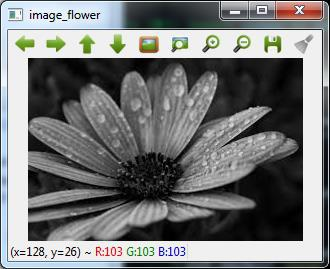Now, we can write the same image into the other format, say .png by using the imwrite() function −

`cv2.imwrite('image_flower.png',image)`

The output True means that the image has been successfully written as .png file also in the same folder.

```True
```

Note − The function destroyallWindows() simply destroys all the windows we created.

### Color Space Conversion

In OpenCV, the images are not stored by using the conventional RGB color, rather they are stored in the reverse order i.e. in the BGR order. Hence the default color code while reading an image is BGR. The cvtColor() color conversion function in for converting the image from one color code to other.

### Example

Consider this example to convert image from BGR to grayscale.

Import the OpenCV package as shown −

`import cv2`

`image = cv2.imread('image_flower.jpg')`

Now, if we see this image using imshow() function, then we can see that this image is in BGR.

`cv2.imshow('BGR_Penguins',image)`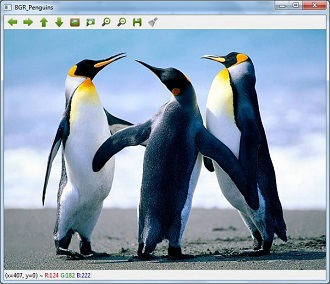Now, use cvtColor() function to convert this image to grayscale.

```image = cv2.cvtColor(image,cv2.COLOR_BGR2GRAY)
cv2.imshow('gray_penguins',image)```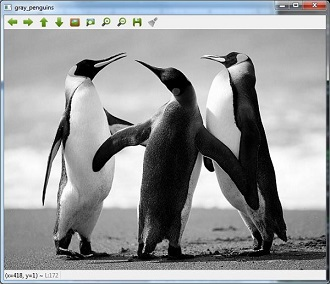### Edge Detection

Humans, after seeing a rough sketch, can easily recognize many object types and their poses. That is why edges play an important role in the life of humans as well as in the applications of computer vision. OpenCV provides very simple and useful function called Canny()for detecting the edges.

### Example

The following example shows clear identification of the edges.

Import OpenCV package as shown −

```import cv2
import numpy as np```

`image = cv2.imread('Penguins.jpg')`

Now, use the Canny () function for detecting the edges of the already read image.

`cv2.imwrite(‘edges_Penguins.jpg’,cv2.Canny(image,200,300))`

Now, for showing the image with edges, use the imshow() function.

`cv2.imshow(‘edges’, cv2.imread(‘‘edges_Penguins.jpg’))`

This Python program will create an image named edges_penguins.jpg with edge detection.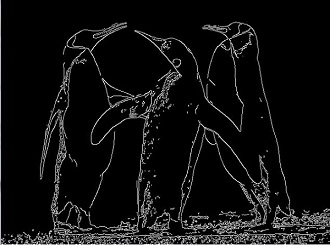### Face Detection

Face detection is one of the fascinating applications of computer vision which makes it more realistic as well as futuristic. OpenCV has a built-in facility to perform face detection. We are going to use the Haar cascade classifier for face detection.

We need data to use the Haar cascade classifier. You can find this data in our OpenCV package. After installing OpenCv, you can see the folder name haarcascades. There would be .xml files for different application. Now, copy all of them for different use and paste then in a new folder under the current project.

Example

The following is the Python code using Haar Cascade to detect the face of Amitabh Bachan shown in the following image −Import the OpenCV package as shown −

```import cv2
import numpy as np```

Now, use the HaarCascadeClassifier for detecting face −

```face_detection=

`img = cv2.imread('AB.jpg')`

Now, convert it into grayscale because it would accept gray images −

`gray = cv2.cvtColor(img, cv2.COLOR_BGR2GRAY)`

Now, using face_detection.detectMultiScale, perform actual face detection

`faces = face_detection.detectMultiScale(gray, 1.3, 5)`

Now, draw a rectangle around the whole face −

```for (x,y,w,h) in faces:
img = cv2.rectangle(img,(x,y),(x+w, y+h),(255,0,0),3)
cv2.imwrite('Face_AB.jpg',img)```

This Python program will create an image named Face_AB.jpg with face detection as shown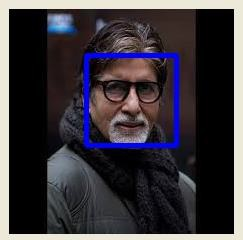### Eye Detection

Eye detection is another fascinating application of computer vision which makes it more realistic as well as futuristic. OpenCV has a built-in facility to perform eye detection. We are going to use the Haar cascade classifier for eye detection.

### Example

The following example gives the Python code using Haar Cascade to detect the face of Amitabh Bachan given in the following image −Import OpenCV package as shown −

```import cv2
import numpy as np```

Now, use the HaarCascadeClassifier for detecting face −

`eye_cascade = cv2.CascadeClassifier('D:/ProgramData/cascadeclassifier/haarcascade_eye.xml')`

`img = cv2.imread('AB_Eye.jpg')`

Now, convert it into grayscale because it would accept grey images −

`gray = cv2.cvtColor(img, cv2.COLOR_BGR2GRAY)`

Now with the help of eye_cascade.detectMultiScale, perform actual face detection

`eyes = eye_cascade.detectMultiScale(gray, 1.03, 5)`

Now, draw a rectangle around the whole face −

```for (ex,ey,ew,eh) in eyes:
img = cv2.rectangle(img,(ex,ey),(ex+ew, ey+eh),(0,255,0),2)
cv2.imwrite('Eye_AB.jpg',img)```

This Python program will create an image named Eye_AB.jpg with eye detection as shown −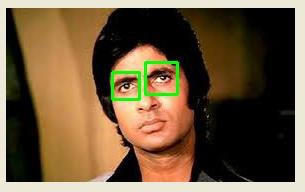## Reinforcement Learning

This type of learning is used to reinforce or strengthen t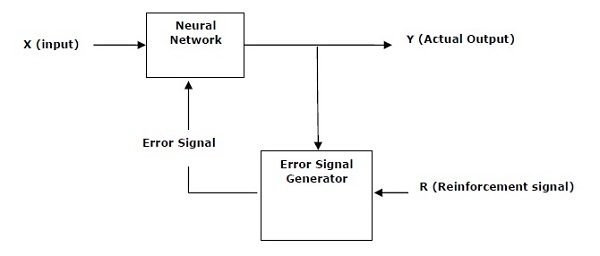he network based on critic information. That is, a network being trained under reinforcement learning, receives some feedback from the environment. However, the feedback is evaluative and not instructive as in the case of supervised learning. Based on this feedback, the network performs the adjustments of the weights to obtain better critic information in future. This learning process is similar to supervised learning but we might have very less information. The following figure gives the block diagram of reinforcement learning.

### Building Blocks: Environment and Agent

Environment and Agent are main building blocks of reinforcement learning in AI. This section discusses them in detail −

### Agent

An agent is anything that can perceive its environment through sensors and acts upon that environment through effectors.

• human agent has sensory organs such as eyes, ears, nose, tongue and skin parallel to the sensors, and other organs such as hands, legs, mouth, for effectors.
• robotic agent replaces cameras and infrared range finders for the sensors, and various motors and actuators for effectors.
• software agent has encoded bit strings as its programs and actions.

### Agent Terminology

The following terms are more frequently used in reinforcement learning in AI −

• Performance Measure of Agent − It is the criteria, which determines how successful an agent is.
• Behavior of Agent − It is the action that agent performs after any given sequence of percepts.
• Percept − It is agent’s perceptual inputs at a given instance.
• Percept Sequence − It is the history of all that an agent has perceived till date.
• Agent Function − It is a map from the precept sequence to an action.

### Environment

• Some programs operate in an entirely artificial environment confined to keyboard input, database, computer file systems and character output on a screen.
• In contrast, some software agents, such as software robots or softbots, exist in rich and unlimited softbot domains. The simulator has a very detailed, and complex environment. The software agent needs to choose from a long array of actions in real time.
• For example, a softbot designed to scan the online preferences of the customer and display interesting items to the customer works in the real as well as an artificial environment.

### Properties of Environment

The environment has multifold properties as discussed below −

• Discrete/Continuous − If there are a limited number of distinct, clearly defined, states of the environment, the environment is discrete , otherwise it is continuous. For example, chess is a discrete environment and driving is a continuous environment.
• Observable/Partially Observable − If it is possible to determine the complete state of the environment at each time point from the percepts, it is observable; otherwise it is only partially observable.
• Static/Dynamic − If the environment does not change while an agent is acting, then it is static; otherwise it is dynamic.
• Single agent/Multiple agents − The environment may contain other agents which may be of the same or different kind as that of the agent.
• Accessible/Inaccessible − If the agent’s sensory apparatus can have acc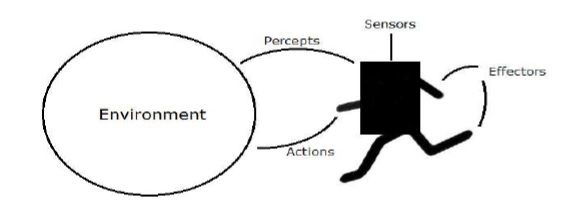ess to the complete state of the environment, then the environment is accessible to that agent; otherwise it is inaccessible.
• Deterministic/Non-deterministic − If the next state of the environment is completely determined by the current state and the actions of the agent, then the environment is deterministic; otherwise it is non-deterministic.
• Episodic/Non-episodic − In an episodic environment, each episode consists of the agent perceiving and then acting. The quality of its action depends just on the episode itself. Subsequent episodes do not depend on the actions in the previous episodes. Episodic environments are much simpler because the agent does not need to think ahead.

### Constructing an Environment with Python

For building reinforcement learning agent, we will be using the OpenAI Gym package which can be installed with the help of the following command −

```pip install gym
```

There are various environments in OpenAI gym which can be used for various purposes. Few of them are Cartpole-v0, Hopper-v1, and MsPacman-v0. They require different engines. The detail documentation of OpenAI Gym can be found on https://gym.openai.com/docs/#environments.

The following code shows an example of Python code for cartpole-v0 environment −

```import gym
env = gym.make('CartPole-v0')
env.reset()
for _ in range(1000):
env.render()
env.step(env.action_space.sample())
```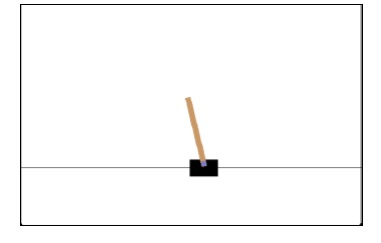You can construct other environments in a similar way.

### Constructing a learning agent with Python

For building reinforcement learning agent, we will be using the OpenAI Gym package as shown −

```import gym
env = gym.make('CartPole-v0')
for _ in range(20):
observation = env.reset()
for i in range(100):
env.render()
print(observation)
action = env.action_space.sample()
observation, reward, done, info = env.step(action)
if done:
print("Episode finished after {} timesteps".format(i+1))
break```Observe that the cartpole can balance itself.

[wpsbx_html_block id=1891]## Speech Recognition

Speech is the most basic means of adult human communication. The basic goal of speech processing is to provide an interaction between a human and a machine.

Speech processing system has mainly three tasks −

• First, speech recognition that allows the machine to catch the words, phrases and sentences we speak
• Second, natural language processing to allow the machine to understand what we speak, and
• Third, speech synthesis to allow the machine to speak.

This chapter focuses on speech recognition, the process of understanding the words that are spoken by human beings. Remember that the speech signals are captured with the help of a microphone and then it has to be understood by the system.

### Building a Speech Recognizer

Speech Recognition or Automatic Speech Recognition (ASR) is the center of attention for AI projects like robotics. Without ASR, it is not possible to imagine a cognitive robot interacting with a human. However, it is not quite easy to build a speech recognizer.

### Difficulties in developing a speech recognition system

Developing a high quality speech recognition system is really a difficult problem. The difficulty of speech recognition technology can be broadly characterized along a number of dimensions as discussed below −

• Size of the vocabulary − Size of the vocabulary impacts the ease of developing an ASR. Consider the following sizes of vocabulary for a better understanding.
• A small size vocabulary consists of 2-100 words, for example, as in a voice-menu system
• A medium size vocabulary consists of several 100s to 1,000s of words, for example, as in a database-retrieval task
• A large size vocabulary consists of several 10,000s of words, as in a general dictation task.

Note that, the larger the size of vocabulary, the harder it is to perform recognition.

• Channel characteristics − Channel quality is also an important dimension. For example, human speech contains high bandwidth with full frequency range, while a telephone speech consists of low bandwidth with limited frequency range. Note that it is harder in the latter.
• Speaking mode − Ease of developing an ASR also depends on the speaking mode, that is whether the speech is in isolated word mode, or connected word mode, or in a continuous speech mode. Note that a continuous speech is harder to recognize.
• Speaking style − A read speech may be in a formal style, or spontaneous and conversational with casual style. The latter is harder to recognize.
• Speaker dependency − Speech can be speaker dependent, speaker adaptive, or speaker independent. A speaker independent is the hardest to build.
• Type of noise − Noise is another factor to consider while developing an ASR. Signal to noise ratio may be in various ranges, depending on the acoustic environment that observes less versus more background noise −
• If the signal to noise ratio is greater than 30dB, it is considered as high range
• If the signal to noise ratio lies between 30dB to 10db, it is considered as medium SNR
• If the signal to noise ratio is lesser than 10dB, it is considered as low range

For example, the type of background noise such as stationary, non-human noise, background speech and crosstalk by other speakers also contributes to the difficulty of the problem.

• Microphone characteristics − The quality of microphone may be good, average, or below average. Also, the distance between mouth and micro-phone can vary. These factors also should be considered for recognition systems.

Despite these difficulties, researchers worked a lot on various aspects of speech such as understanding the speech signal, the speaker, and identifying the accents.

You will have to follow the steps given below to build a speech recognizer:

### Visualizing Audio Signals:

Reading from a File and Working on it. This is the first step in building speech recognition system as it gives an understanding of how an audio signal is structured. Some common steps that can be followed to work with audio signals are as follows −

### Recording

When you have to read the audio signal from a file, then record it using a microphone, at first.

### Sampling

When recording with microphone, the signals are stored in a digitized form. But to work upon it, the machine needs them in the discrete numeric form. Hence, we should perform sampling at a certain frequency and convert the signal into the discrete numerical form. Choosing the high frequency for sampling implies that when humans listen to the signal, they feel it as a continuous audio signal.

### Example

The following example shows a stepwise approach to analyze an audio signal, using Python, which is stored in a file. The frequency of this audio signal is 44,100 HZ.

Import the necessary packages as shown here −

```import numpy as np
import matplotlib.pyplot as plt
from scipy.io import wavfile```

Now, read the stored audio file. It will return two values: the sampling frequency and the audio signal. Provide the path of the audio file where it is stored, as shown here −

`frequency_sampling, audio_signal = wavfile.read("/Users/admin/audio_file.wav")`

Display the parameters like sampling frequency of the audio signal, data type of signal and its duration, using the commands shown −

```print('\nSignal shape:', audio_signal.shape)
print('Signal Datatype:', audio_signal.dtype)
print('Signal duration:', round(audio_signal.shape /
float(frequency_sampling), 2), 'seconds')```

This step involves normalizing the signal as shown below −

`audio_signal = audio_signal / np.power(2, 15)`

In this step, we are extracting the first 100 values from this signal to visualize. Use the following commands for this purpose −

```audio_signal = audio_signal [:100]
time_axis = 1000 * np.arange(0, len(signal), 1) / float(frequency_sampling)
```

Now, visualize the signal using the commands given below −

```plt.plot(time_axis, signal, color='blue')
plt.xlabel('Time (milliseconds)')
plt.ylabel('Amplitude')
plt.title('Input audio signal')
plt.show()```

You would be able to see an output graph and data extracted for the above audio signal as shown in the image here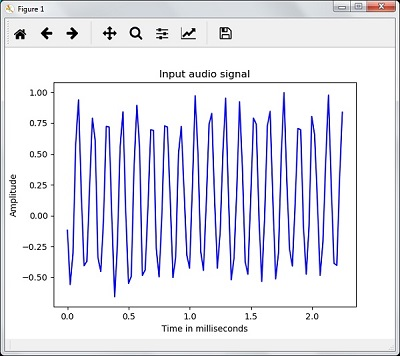```Signal shape: (132300,)
Signal Datatype: int16
Signal duration: 3.0 seconds```

### Characterizing the Audio Signal: Transforming to Frequency Domain

Characterizing an audio signal involves converting the time domain signal into frequency domain, and understanding its frequency components, by. This is an important step because it gives a lot of information about the signal. You can use a mathematical tool like Fourier Transform to perform this transformation.

### Example

The following example shows, step-by-step, how to characterize the signal, using Python, which is stored in a file. Note that here we are using Fourier Transform mathematical tool to convert it into frequency domain.

Import the necessary packages, as shown here −

```import numpy as np
import matplotlib.pyplot as plt
from scipy.io import wavfile```

Now, read the stored audio file. It will return two values: the sampling frequency and the the audio signal. Provide the path of the audio file where it is stored as shown in the command here −

```frequency_sampling, audio_signal = wavfile.read("/Users/admin/sample.wav")
```

In this step, we will display the parameters like sampling frequency of the audio signal, data type of signal and its duration, using the commands given below −

```print('\nSignal shape:', audio_signal.shape)
print('Signal Datatype:', audio_signal.dtype)
print('Signal duration:', round(audio_signal.shape /
float(frequency_sampling), 2), 'seconds')```

In this step, we need to normalize the signal, as shown in the following command −

```audio_signal = audio_signal / np.power(2, 15)
```

This step involves extracting the length and half length of the signal. Use the following commands for this purpose −

```length_signal = len(audio_signal)
half_length = np.ceil((length_signal + 1) / 2.0).astype(np.int)
```

Now, we need to apply mathematics tools for transforming into frequency domain. Here we are using the Fourier Transform.

`signal_frequency = np.fft.fft(audio_signal)`

Now, do the normalization of frequency domain signal and square it −

```signal_frequency = abs(signal_frequency[0:half_length]) / length_signal
signal_frequency **= 2```

Next, extract the length and half length of the frequency transformed signal −

`len_fts = len(signal_frequency)`

Note that the Fourier transformed signal must be adjusted for even as well as odd case.

```if length_signal % 2:
signal_frequency[1:len_fts] *= 2
else:
signal_frequency[1:len_fts-1] *= 2```

Now, extract the power in decibal(dB) −

`signal_power = 10 * np.log10(signal_frequency)`

Adjust the frequency in kHz for X-axis −

`x_axis = np.arange(0, len_half, 1) * (frequency_sampling / length_signal) / 1000.0`

Now, visualize the characterization of signal as follows −

```plt.figure()
plt.plot(x_axis, signal_power, color='black')
plt.xlabel('Frequency (kHz)')
plt.ylabel('Signal power (dB)')
plt.show()```

You can observe the output graph of the above code as shown in the image below −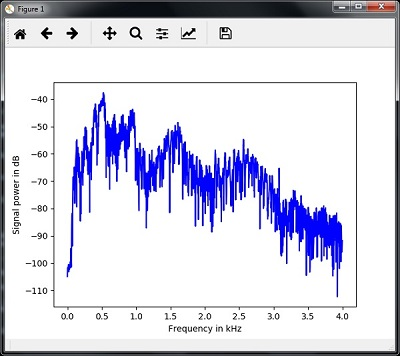### Generating Monotone Audio Signal

The two steps that you have seen till now are important to learn about signals. Now, this step will be useful if you want to generate the audio signal with some predefined parameters. Note that this step will save the audio signal in an output file.

### Example

In the following example, we are going to generate a monotone signal, using Python, which will be stored in a file. For this, you will have to take the following steps −

Import the necessary packages as shown −

```import numpy as np
import matplotlib.pyplot as plt
from scipy.io.wavfile import write```

Provide the file where the output file should be saved

```output_file = 'audio_signal_generated.wav'
```

Now, specify the parameters of your choice, as shown −

```duration = 4 # in seconds
frequency_sampling = 44100 # in Hz
frequency_tone = 784
min_val = -4 * np.pi
max_val = 4 * np.pi```

In this step, we can generate the audio signal, as shown −

```t = np.linspace(min_val, max_val, duration * frequency_sampling)
audio_signal = np.sin(2 * np.pi * tone_freq * t)```

Now, save the audio file in the output file −

```write(output_file, frequency_sampling, signal_scaled)
```

Extract the first 100 values for our graph, as shown −

```audio_signal = audio_signal[:100]
time_axis = 1000 * np.arange(0, len(signal), 1) / float(sampling_freq)```

Now, visualize the generated audio signal as follows −

```plt.plot(time_axis, signal, color='blue')
plt.xlabel('Time in milliseconds')
plt.ylabel('Amplitude')
plt.title('Generated audio signal')
plt.show()```

You can observe the plot as shown in the figure given here −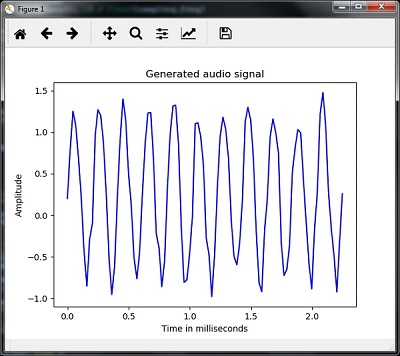### Feature Extraction from Speech

This is the most important step in building a speech recognizer because after converting the speech signal into the frequency domain, we must convert it into the usable form of feature vector. We can use different feature extraction techniques like MFCC, PLP, PLP-RASTA etc. for this purpose.

### Example

In the following example, we are going to extract the features from signal, step-by-step, using Python, by using MFCC technique.

Import the necessary packages, as shown here −

```import numpy as np
import matplotlib.pyplot as plt
from scipy.io import wavfile
from python_speech_features import mfcc, logfbank```

Now, read the stored audio file. It will return two values − the sampling frequency and the audio signal. Provide the path of the audio file where it is stored.

`frequency_sampling, audio_signal = wavfile.read("/Users/admin/audio_file.wav")`

Note that here we are taking first 15000 samples for analysis.

`audio_signal = audio_signal[:15000]`

Use the MFCC techniques and execute the following command to extract the MFCC features −

```features_mfcc = mfcc(audio_signal, frequency_sampling)
```

Now, print the MFCC parameters, as shown −

```print('\nMFCC:\nNumber of windows =', features_mfcc.shape)
print('Length of each feature =', features_mfcc.shape)```

Now, plot and visualize the MFCC features using the commands given below −

```features_mfcc = features_mfcc.T
plt.matshow(features_mfcc)
plt.title('MFCC')```

In this step, we work with the filter bank features as shown −

Extract the filter bank features −

`filterbank_features = logfbank(audio_signal, frequency_sampling)`

Now, print the filterbank parameters.

```print('\nFilter bank:\nNumber of windows =', filterbank_features.shape)
print('Length of each feature =', filterbank_features.shape)```

Now, plot and visualize the filterbank features.

```filterbank_features = filterbank_features.T
plt.matshow(filterbank_features)
plt.title('Filter bank')
plt.show()```

As a result of the steps above, you can observe the following outputs: Figure1 for MFCC and Figure2 for Filter Bank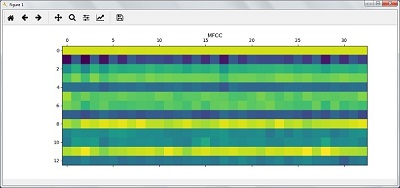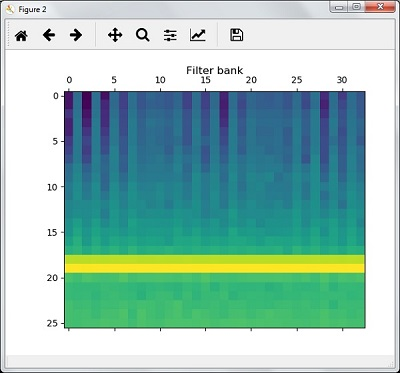### Recognition of Spoken Words

Speech recognition means that when humans are speaking, a machine understands it. Here we are using Google Speech API in Python to make it happen. We need to install the following packages for this −

• Pyaudio − It can be installed by using pip install Pyaudio command.
• SpeechRecognition − This package can be installed by using pip install SpeechRecognition.
• Google-Speech-API − It can be installed by using the command pip install google-api-python-client.

### Example

Observe the following example to understand about recognition of spoken words −

Import the necessary packages as shown −

`import speech_recognition as sr`

Create an object as shown below −

`recording = sr.Recognizer()`

Now, the Microphone() module will take the voice as input −

```with sr.Microphone() as source: recording.adjust_for_ambient_noise(source)
audio = recording.listen(source)```

Now google API would recognize the voice and gives the output.

```try:
except Exception as e:
print(e)
```

You can see the following output −

```Please Say Something:
You said:
```

For example, if you said tutorialspoint.com, then the system recognizes it correctly as follows −

`tutorialspoint.com`

[wpsbx_html_block id=1891]## Supervised Learning: Regression

Regression is one of the most important statistical and machine learning tools. We would not be wrong to say that the journey of machine learning starts from regression. It may be defined as the parametric technique that allows us to make decisions based upon data or in other words allows us to make predictions based upon data by learning the relationship between input and output variables. Here, the output variables dependent on the input variables, are continuous-valued real numbers. In regression, the relationship between input and output variables matters and it helps us in understanding how the value of the output variable changes with the change of input variable. Regression is frequently used for prediction of prices, economics, variations, and so on.

### Building Regressors in Python

In this section, we will learn how to build single as well as multivariable regressor.

### Linear Regressor/Single Variable Regressor

Let us important a few required packages −

```import numpy as np
from sklearn import linear_model
import sklearn.metrics as sm
import matplotlib.pyplot as plt```

Now, we need to provide the input data and we have saved our data in the file named linear.txt.

`input = 'D:/ProgramData/linear.txt'`

```input_data = np.loadtxt(input, delimiter=',')
X, y = input_data[:, :-1], input_data[:, -1]```

The next step would be to train the model. Let us give training and testing samples.

```training_samples = int(0.6 * len(X))
testing_samples = len(X) - num_training

X_train, y_train = X[:training_samples], y[:training_samples]

X_test, y_test = X[training_samples:], y[training_samples:]```

Now, we need to create a linear regressor object.

`reg_linear = linear_model.LinearRegression()`

Train the object with the training samples.

`reg_linear.fit(X_train, y_train)`

We need to do the prediction with the testing data.

`y_test_pred = reg_linear.predict(X_test)`

Now plot and visualize the data.

```plt.scatter(X_test, y_test, color = 'red')
plt.plot(X_test, y_test_pred, color = 'black', linewidth = 2)
plt.xticks(())
plt.yticks(())
plt.show()```

### Output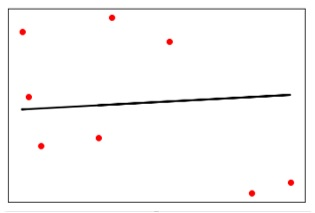Now, we can compute the performance of our linear regression as follows −

```print("Performance of Linear regressor:")
print("Mean absolute error =", round(sm.mean_absolute_error(y_test, y_test_pred), 2))
print("Mean squared error =", round(sm.mean_squared_error(y_test, y_test_pred), 2))
print("Median absolute error =", round(sm.median_absolute_error(y_test, y_test_pred), 2))
print("Explain variance score =", round(sm.explained_variance_score(y_test, y_test_pred),
2))
print("R2 score =", round(sm.r2_score(y_test, y_test_pred), 2))```

### Output

Performance of Linear Regressor −

```Mean absolute error = 1.78
Mean squared error = 3.89
Median absolute error = 2.01
Explain variance score = -0.09
R2 score = -0.09
```

In the above code, we have used this small data. If you want some big dataset then you can use sklearn.dataset to import bigger dataset.

```2,4.82.9,4.72.5,53.2,5.56,57.6,43.2,0.92.9,1.92.4,
3.50.5,3.41,40.9,5.91.2,2.583.2,5.65.1,1.54.5,
1.22.3,6.32.1,2.8```

### Multivariable Regressor

First, let us import a few required packages −

```import numpy as np
from sklearn import linear_model
import sklearn.metrics as sm
import matplotlib.pyplot as plt
from sklearn.preprocessing import PolynomialFeatures```

Now, we need to provide the input data and we have saved our data in the file named linear.txt.

`input = 'D:/ProgramData/Mul_linear.txt'`

```input_data = np.loadtxt(input, delimiter=',')
X, y = input_data[:, :-1], input_data[:, -1]```

The next step would be to train the model; we will give training and testing samples.

```training_samples = int(0.6 * len(X))
testing_samples = len(X) - num_training

X_train, y_train = X[:training_samples], y[:training_samples]

X_test, y_test = X[training_samples:], y[training_samples:]```

Now, we need to create a linear regressor object.

`reg_linear_mul = linear_model.LinearRegression()`

Train the object with the training samples.

`reg_linear_mul.fit(X_train, y_train)`

Now, at last we need to do the prediction with the testing data.

```y_test_pred = reg_linear_mul.predict(X_test)

print("Performance of Linear regressor:")
print("Mean absolute error =", round(sm.mean_absolute_error(y_test, y_test_pred), 2))
print("Mean squared error =", round(sm.mean_squared_error(y_test, y_test_pred), 2))
print("Median absolute error =", round(sm.median_absolute_error(y_test, y_test_pred), 2))
print("Explain variance score =", round(sm.explained_variance_score(y_test, y_test_pred), 2))
print("R2 score =", round(sm.r2_score(y_test, y_test_pred), 2))
```

### Output

Performance of Linear Regressor −

```Mean absolute error = 0.6
Mean squared error = 0.65
Median absolute error = 0.41
Explain variance score = 0.34
R2 score = 0.33
```

Now, we will create a polynomial of degree 10 and train the regressor. We will provide the sample data point.

```polynomial = PolynomialFeatures(degree = 10)
X_train_transformed = polynomial.fit_transform(X_train)
datapoint = [[2.23, 1.35, 1.12]]
poly_datapoint = polynomial.fit_transform(datapoint)

poly_linear_model = linear_model.LinearRegression()
poly_linear_model.fit(X_train_transformed, y_train)
print("\nLinear regression:\n", reg_linear_mul.predict(datapoint))
print("\nPolynomial regression:\n", poly_linear_model.predict(poly_datapoint))```

### Output

Linear regression:

```[2.40170462]
```

Polynomial regression:

```[1.8697225]
```

In the above code, we have used this small data. If you want a big dataset then, you can use sklearn.dataset to import a bigger dataset.

```2,4.8,1.2,3.22.9,4.7,1.5,3.62.5,5,2.8,23.2,5.5,3.5,2.16,5,
2,3.27.6,4,1.2,3.23.2,0.9,2.3,1.42.9,1.9,2.3,1.22.4,3.5,
2.8,3.60.5,3.4,1.8,2.91,4,3,2.50.9,5.9,5.6,0.81.2,2.58,
3.45,1.233.2,5.6,2,3.25.1,1.5,1.2,1.34.5,1.2,4.1,2.32.3,
6.3,2.5,3.22.1,2.8,1.2,3.6```

[wpsbx_html_block id=1891]## Artificial Neural Networks (ANNs)

An artificial neuron receives a signal then processes it and can signal neurons connected to it. The inventor of the first neurocomputer, Dr. Robert Hecht-Nielsen, defines a neural network as a computing system made up of a number of simple, highly interconnected processing elements, which process information by their dynamic state response to external inputs.

### Basic Structure of ANNs

The idea of ANNs is based on the belief that working of human brain by making the right connections, can be imitated using silicon and wires as living neurons and dendrites. The human brain is composed of 86 billion nerve cells called neurons. They are connected to other thousand cells by Axons. Stimuli from external environment or inputs from sensory organs are accepted by dendrites. These inputs create electric impulses, which quickly travel through the neural network. A neuron can then send the message to other neuron to handle the issue or does not send it forward.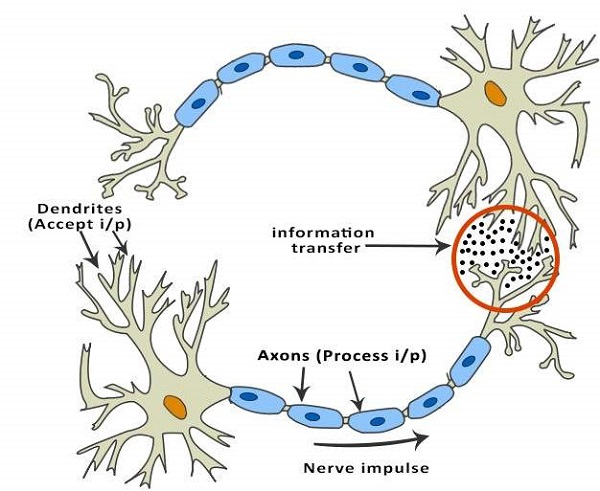ANNs are composed of multiple nodes, which imitate biological neurons of human brain. The neurons are connected by links and they interact with each other. The nodes can take input data and perform simple operations on the data. The result of these operations is passed to other neurons. The output at each node is called its activation or node value. Each link is associated with weight. ANNs are capable of learning, which takes place by altering weight values. The following illustration shows a simple ANN −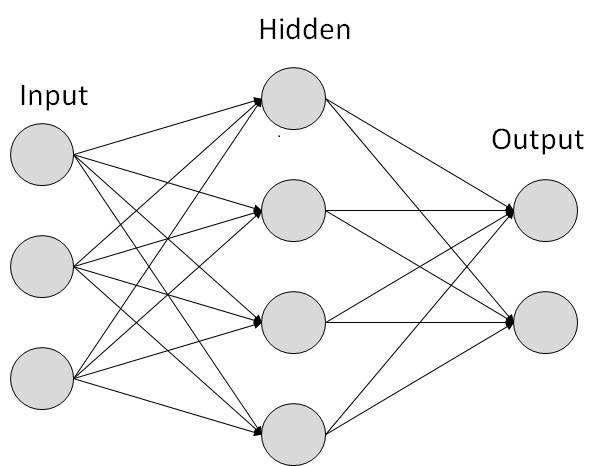### Types of Artificial Neural Networks

There are two Artificial Neural Network topologies; FeedForward and Feedback.

### FeedForward ANN

In this ANN, the information flow is unidirectional. A unit sends information to other unit from which it does not receive any information. There are no feedback loops. They are used in pattern generation/recognition/classification. They have fixed inputs and outputs.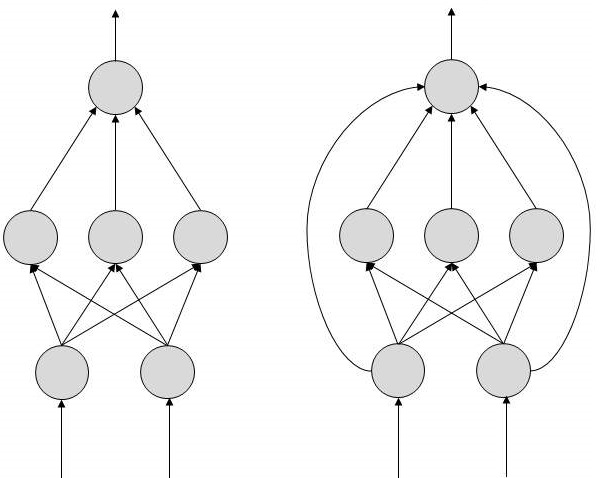### FeedBack ANN

Here, feedback loops are allowed. They are used in content addressable memories.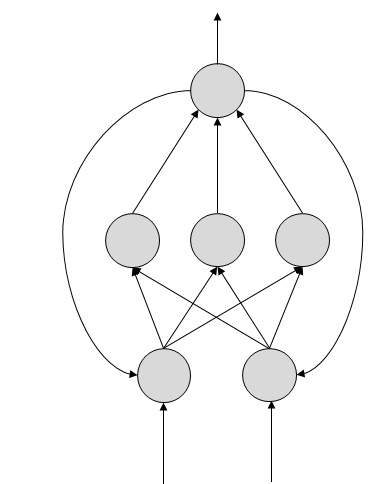### Working of ANNs

In the topology diagrams shown, each arrow represents a connection between two neurons and indicates the pathway for the flow of information. Each connection has a weight, an integer number that controls the signal between the two neurons. If the network generates a “good or desired” output, there is no need to adjust the weights. However, if the network generates a “poor or undesired” output or an error, then the system alters the weights in order to improve subsequent results.

[wpsbx_html_block id=1891]

### Machine Learning in ANNs

ANNs are capable of learning and they need to be trained. There are several learning strategies −

• Supervised Learning − It involves a teacher that is scholar than the ANN itself. For example, the teacher feeds some example data about which the teacher already knows the answers.For example, pattern recognizing. The ANN comes up with guesses while recognizing. Then the teacher provides the ANN with the answers. The network then compares it guesses with the teacher’s “correct” answers and makes adjustments according to errors.
• Unsupervised Learning − It is required when there is no example data set with known answers. For example, searching for a hidden pattern. In this case, clustering i.e. dividing a set of elements into groups according to some unknown pattern is carried out based on the existing data sets present.
• Reinforcement Learning − This strategy built on observation. The ANN makes a decision by observing its environment. If the observation is negative, the network adjusts its weights to be able to make a different required decision the next time.

### Back Propagation Algorithm

It is the training or learning algorithm. It learns by example. If you submit to the algorithm the example of what you want the network to do, it changes the network’s weights so that it can produce desired output for a particular input on finishing the training. Back Propagation networks are ideal for simple Pattern Recognition and Mapping Tasks.

### Bayesian Networks (BN)

• These are the graphical structures used to represent the probabilistic relationship among a set of random variables. Bayesian networks are also called Belief Networks or Bayes Nets. BNs reason about uncertain domain.
• In these networks, each node represents a random variable with specific propositions. For example, in a medical diagnosis domain, the node Cancer represents the proposition that a patient has cancer.
• The edges connecting the nodes represent probabilistic dependencies among those random variables. If out of two nodes, one is affecting the other then they must be directly connected in the directions of the effect. The strength of the relationship between variables is quantified by the probability associated with each node.
• There is an only constraint on the arcs in a BN that you cannot return to a node simply by following directed arcs. Hence the BNs are called Directed Acyclic Graphs (DAGs).

BNs are capable of handling multivalued variables simultaneously. The BN variables are composed of two dimensions −

• Range of prepositions
• Probability assigned to each of the prepositions.

Consider a finite set X = {X1, X2, …,Xn} of discrete random variables, where each variable Xi may take values from a finite set, denoted by Val(Xi). If there is a directed link from variable Xi to variable, Xj, then variable Xi will be a parent of variable Xj showing direct dependencies between the variables.

The structure of BN is ideal for combining prior knowledge and observed data. BN can be used to learn the causal relationships and understand various problem domains and to predict future events, even in case of missing data.

### Building a Bayesian Network

A knowledge engineer can build a Bayesian network. There are a number of steps the knowledge engineer needs to take while building it.

Example problem − Lung cancer. A patient has been suffering from breathlessness. He visits the doctor, suspecting he has lung cancer. The doctor knows that barring lung cancer, there are various other possible diseases the patient might have such as tuberculosis and bronchitis.

Gather Relevant Information of Problem

• Is the patient a smoker? If yes, then high chances of cancer and bronchitis.
• Is the patient exposed to air pollution? If yes, what sort of air pollution?
• Take an X-Ray positive X-ray would indicate either TB or lung cancer.

Identify Interesting Variables

The knowledge engineer tries to answer the questions −

• Which nodes to represent?
• What values can they take? In which state can they be?

For now let us consider nodes, with only discrete values. The variable must take on exactly one of these values at a time.

Common types of discrete nodes are:

• Boolean nodes − They represent propositions, taking binary values TRUE (T) and FALSE (F).
• Ordered values − A node Pollution might represent and take values from {low, medium, high} describing degree of a patient’s exposure to pollution.
• Integral values − A node called Age might represent patient’s age with possible values from 1 to 120. Even at this early stage, modeling choices are being made.

Possible nodes and values for the lung cancer example −

Node Name Type Value Nodes Creation
Polution Binary {LOW, HIGH, MEDIUM}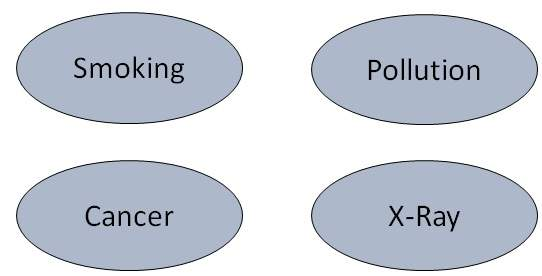Smoker Boolean {TRUE, FASLE}
Lung-Cancer Boolean {TRUE, FASLE}
X-Ray Binary {Positive, Negative}

Create Arcs between Nodes

• Topology of the network should capture qualitative relationships between variables.
• For example, what causes a patient to have lung cancer? – Pollution and smoking. Then add arcs from node Pollution and node Smoker to node Lung-Cancer.
• Similarly if patient has lung cancer, then X-ray result will be positive. Then add arcs from node Lung-Cancer to node X-Ray.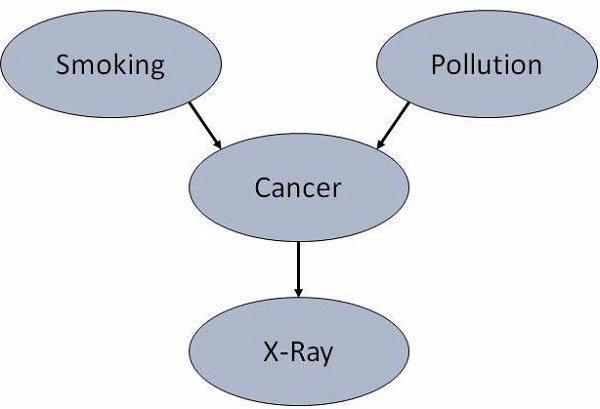Specify Topology

• Conventionally, BNs are laid out so that the arcs point from top to bottom. The set of parent nodes of a node X is given by Parents(X).
• The Lung-Cancer node has two parents (reasons or causes): Pollution and Smoker, while node Smoker is an ancestor of node X-Ray. Similarly, X-Ray is a child (consequence or effects) of node Lung-Cancer and successor of nodes Smoker and Pollution.
• Conditional Probabilities
• Now quantify the relationships between connected nodes: this is done by specifying a conditional probability distribution for each node. As only discrete variables are considered here, this takes the form of a Conditional Probability Table (CPT).
• First, for each node we need to look at all the possible combinations of values of those parent nodes. Each such combination is called an instantiation of the parent set. For each distinct instantiation of parent node values, we need to specify the probability that the child will take.

For example, the Lung-Cancer node’s parents are Pollution and Smoking. They take the possible values = { (H,T), ( H,F), (L,T), (L,F)}. The CPT specifies the probability of cancer for each of these cases as <0.05, 0.02, 0.03, 0.001> respectively.

Each node will have conditional probability associated as follows −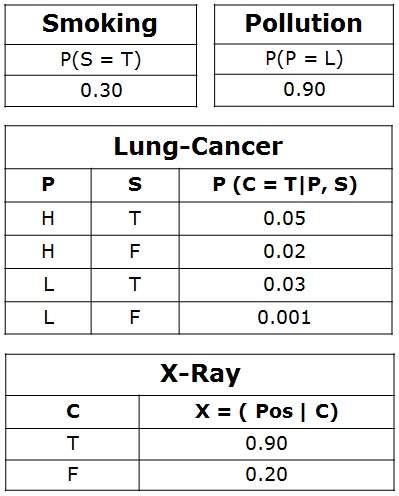### Applications of Neural Networks

They can perform tasks that are easy for a human but difficult for a machine −

• Aerospace − Autopilot aircrafts, aircraft fault detection.
• Automotive − Automobile guidance systems.
• Military − Weapon orientation and steering, target tracking, object discrimination, facial recognition, signal/image identification.
• Electronics − Code sequence prediction, IC chip layout, chip failure analysis, machine vision, voice synthesis.
• Financial − Real estate appraisal, loan advisor, mortgage screening, corporate bond rating, portfolio trading program, corporate financial analysis, currency value prediction, document readers, credit application evaluators.
• Industrial − Manufacturing process control, product design and analysis, quality inspection systems, welding quality analysis, paper quality prediction, chemical product design analysis, dynamic modeling of chemical process systems, machine maintenance analysis, project bidding, planning, and management.
• Medical − Cancer cell analysis, EEG and ECG analysis, prosthetic design, transplant time optimizer.
• Speech − Speech recognition, speech classification, text to speech conversion.
• Telecommunications − Image and data compression, automated information services, real-time spoken language translation.
• Transportation − Truck Brake system diagnosis, vehicle scheduling, routing systems.
• Software − Pattern Recognition in facial recognition, optical character recognition, etc.
• Time Series Prediction − ANNs are used to make predictions on stocks and natural calamities.
• Signal Processing − Neural networks can be trained to process an audio signal and filter it appropriately in the hearing aids.
• Control − ANNs are often used to make steering decisions of physical vehicles.
• Anomaly Detection − As ANNs are expert at recognizing patterns, they can also be trained to generate an output when something unusual occurs that misfits the pattern.

### Threat to Privacy

An AI program that recognizes speech and understands natural language is theoretically capable of understanding each conversation on e-mails and telephones.

### Threat to Human Dignity

AI systems have already started replacing the human beings in few industries. It should not replace people in the sectors where they are holding dignified positions which are pertaining to ethics such as nursing, surgeon, judge, police officer, etc.

### Threat to Safety

The self-improving AI systems can become so mighty than humans that could be very difficult to stop from achieving their goals, which may lead to unintended consequences.

Here is the list of frequently used terms in the domain of AI −

Sr.No Term & Meaning
1 Agent

Agents are systems or software programs capable of autonomous, purposeful and reasoning directed towards one or more goals. They are also called assistants, brokers, bots, droids, intelligent agents, and software agents.

2 Autonomous Robot

Robot free from external control or influence and able to control itself independently.

3 Backward Chaining

Strategy of working backward for Reason/Cause of a problem.

4 Blackboard

It is the memory inside computer, which is used for communication between the cooperating expert systems.

5 Environment

It is the part of real or computational world inhabited by the agent.

6 Forward Chaining

Strategy of working forward for conclusion/solution of a problem.

7 Heuristics

It is the knowledge based on Trial-and-error, evaluations, and experimentation.

8 Knowledge Engineering

Acquiring knowledge from human experts and other resources.

9 Percepts

It is the format in which the agent obtains information about the environment.

10 Pruning

Overriding unnecessary and irrelevant considerations in AI systems.

11 Rule

It is a format of representing knowledge base in Expert System. It is in the form of IF-THEN-ELSE.

12 Shell

A shell is a software that helps in designing inference engine, knowledge base, and user interface of an expert system.

It is the goal the agent is tries to accomplish.

14 Turing Test

A test developed by Allan Turing to test the intelligence of a machine as compared to human intelligence.## Part 2: Artificial Intelligence research areas and Agent

Artificial Intelligence research areas and Agent are discussed in the article. Here firstly we are going to know about speech and voice recognition.

## Speech and Voice Recognition

These both terms are common in robotics, expert systems and natural language processing. Though these terms are used interchangeably, their objectives are different.

Speech Recognition Voice Recognition
The speech recognition aims at understanding and comprehending WHAT was spoken. The objective of voice recognition is to recognize WHO is speaking.
It is used in hand-free computing, map, or menu navigation. It is used to identify a person by analyzing its tone, voice pitch, and accent, etc.
Machine does not need training for Speech Recognition as it is not speaker dependent. This recognition system needs training as it is person oriented.
Speaker independent Speech Recognition systems are difficult to develop. Speaker dependent Speech Recognition systems are comparatively easy to develop.

### Working of (Speech and Voice) Recognition Systems

The user input spoken at a microphone goes to sound card of the system. The converter turns the analog signal into equivalent digital signal for the speech processing. The database is used to compare the sound patterns to recognize the words. Finally, a reverse feedback is given to the database. This source-language text becomes input to the Translation Engine, which converts it to the target language text. They are supported with interactive GUI, large database of vocabulary, etc.

### Real Life Applications of Research Areas

There is a large array of applications where AI is serving common people in their day-to-day lives:

Expert Systems

Examples − Flight-tracking systems, Clinical systems.

Natural Language Processing

Examples: Google Now feature, speech recognition, Automatic voice output.

Neural Networks

Examples − Pattern recognition systems such as face recognition, character recognition, handwriting recognition.

Robotics

Examples − Industrial robots for moving, spraying, painting, precision checking, drilling, cleaning, coating, carving, etc.

Fuzzy Logic Systems

Examples − Consumer electronics, automobiles, microwave oven etc.

Perception

• Computer Vision
• Speech, Voice
• Mathematics
• Geometry
• Logic
• Integration and Differentiation
• Engineering
• Fault Finding
• Manufacturing
• Monitoring
Natural Language Processing

• Understanding
• Language Generation
• Language Translation
Games

• Go
• Chess (Deep Blue)
• Ckeckers
Scientific Analysis
Common Sense Verification Financial Analysis
Reasoning Theorem Proving Medical Diagnosis
Planing Creativity
Robotics

• Locomotive

[wpsbx_html_block id=1891]

Humans learn ordinary (mundane ) tasks since their birth. They learn by perception, speaking, using language, and locomotives. They learn Formal Tasks and Expert Tasks later, in that order. For humans, the mundane tasks are easiest to learn. The same was considered true before trying to implement mundane tasks in machines. Earlier, all work of AI was concentrated in the mundane task domain. Later, it turned out that the machine requires more knowledge, complex knowledge representation, and complicated algorithms for handling mundane tasks. This is the reason why AI work is more prospering in the Expert Tasks domain now, as the expert task domain needs expert knowledge without common sense, which can be easier to represent and handle.

### Agent and Environment:

An agent is anything that can perceive its environment through sensors and acts upon that environment through effectors.

1. human agent has sensory organs such as eyes, ears, nose, tongue and skin parallel to the sensors, and other organs such as hands, legs, mouth, for effectors.
2. robotic agent replaces cameras and infrared range finders for the sensors, and various motors and actuators for effectors.
3. software agent has encoded bit strings as its programs and actions.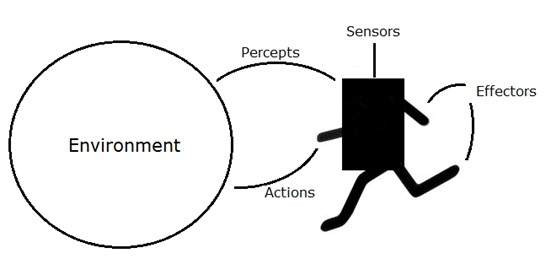### Agent Terminology

1. Performance Measure of Agent: It is the criteria, which determines how successful an agent is.
2. Behavior of Agent: It is the action that agent performs after any given sequence of percepts.
3. Percept: It is agent’s perceptual inputs at a given instance.
4. Percept Sequence: It is the history of all that an agent has perceived till date.
5. Agent Function: It is a map from the precept sequence to an action.

### Rationality

Rationality is a status of being reasonable, sensible, and having good sense of judgment. It is concerned with expected actions and results depending upon what the agent has perceived. Performing actions with the aim of obtaining useful information is an important part of rationality.

### Ideal Rational Agent:

An ideal rational agent is the one, which is capable of doing expected actions to maximize its performance measure, on the basis of −

1. Its percept sequence
2. Its built-in knowledge base

Rationality of an agent depends on the following −

1. The performance measures, which determine the degree of success.
2. Agent’s Percept Sequence till now.
3. The agent’s prior knowledge about the environment.
4. The actions that the agent can carry out.

A rational agent always performs right action, where the right action means the action that causes the agent to be most successful in the given percept sequence. The problem the agent solves is characterized by Performance Measure, Environment, Actuators, and Sensors (PEAS).

### The Structure of Intelligent Agents

Agent’s structure can be viewed as:

1. Agent = Architecture + Agent Program
2. Architecture = the machinery that an agent executes on.
3. Agent Program = an implementation of an agent function.

### Simple Reflex Agents

• They choose actions only based on the current percept.
• They are rational only if a correct decision is made only on the basis of current precept.
• Their environment is completely observable.

Condition-Action Rule − It is a rule that maps a state (condition) to an action.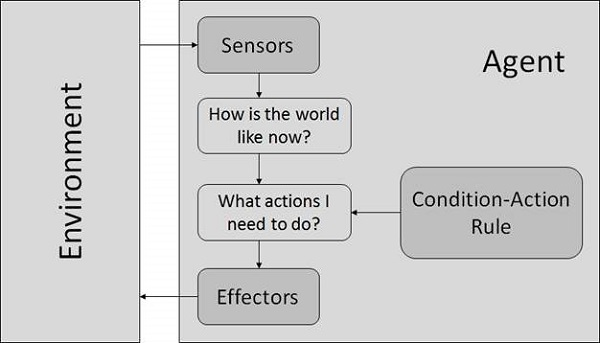### Model Based Reflex Agents

They use a model of the world to choose their actions. They maintain an internal state.

Model − knowledge about “how the things happen in the world”.

Internal State − It is a representation of unobserved aspects of current state depending on percept history.

Updating the state requires the information about:

• How the world evolves.
• How the agent’s actions affect the world.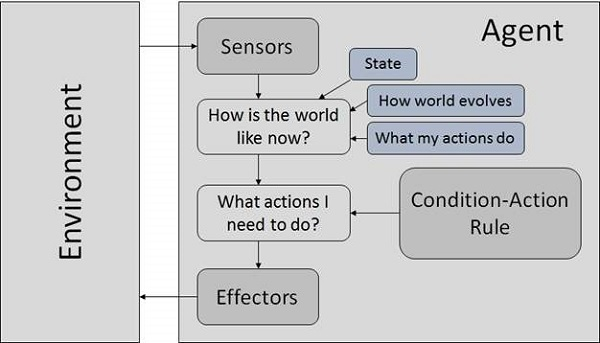### Goal Based Agents

They choose their actions in order to achieve goals. Goal-based approach is more flexible than reflex agent since the knowledge supporting a decision is explicitly modeled, thereby allowing for modifications.

Goal − It is the description of desirable situations.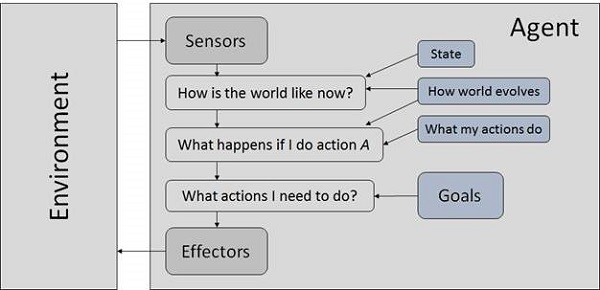### Utility Based Agents

They choose actions based on a preference (utility) for each state.

• There are conflicting goals, out of which only few can be achieved.
• Goals have some uncertainty of being achieved and you need to weigh likelihood of success against the importance of a goal.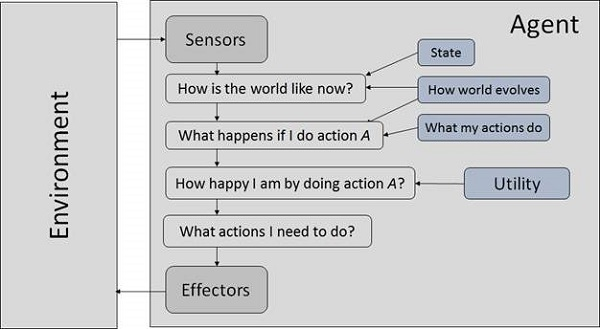### The Nature of Environments

Some programs operate in the entirely artificial environment confined to keyboard input, database, computer file systems and character output on a screen. Besides, some software agents (software robots or softbots) exist in rich, unlimited softbots domains. The simulator has a very detailed, complex environment. The software agent needs to choose from a long array of actions in real time. A softbot designed to scan the online preferences of the customer and show interesting items to the customer works in the real as well as an artificial environment. The most famous artificial environment is the Turing Test environment, in which one real and other artificial agents are tested on equal ground. This is a very challenging environment as it is highly difficult for a software agent to perform as well as a human.

### Turing Test: The success of an intelligent behavior of a system can be measured with Turing Test.

Two persons and a machine to be evaluated participate in the test. Out of the two persons, one plays the role of the tester. Each of them sits in different rooms. The tester is unaware of who is machine and who is a human. He interrogates the questions by typing and sending them to both intelligences, to which he receives typed responses. This test aims at fooling the tester. If the tester fails to determine machine’s response from the human response, then the machine is said to be intelligent.

### Properties of Environment

The environment has multifold properties: Discrete / Continuous − If there are a limited number of distinct, clearly defined, states of the environment, the environment is discrete (For example, chess); otherwise it is continuous (For example, driving).

1. Observable / Partially Observable: If it is possible to determine the complete state of the environment at each time point from the percepts it is observable; otherwise it is only partially observable.
2. Static / Dynamic: If the environment does not change while an agent is acting, then it is static; otherwise it is dynamic.
3. Single agent / Multiple agents: The environment may contain other agents which may be of the same or different kind as that of the agent.
4. Accessible / Inaccessible: If the agent’s sensory apparatus can have access to the complete state of the environment, then the environment is accessible to that agent.
5. Deterministic / Non-deterministic: If the next state of the environment is completely determined by the current state and the actions of the agent, then the environment is deterministic; otherwise it is non-deterministic.
6. Episodic / Non-episodic: In an episodic environment, each episode consists of the agent perceiving and then acting. The quality of its action depends just on the episode itself. Subsequent episodes do not depend on the actions in the previous episodes. Episodic environments are much simpler because the agent does not need to think ahead.## What is Artificial Intelligence?

AI has the potential to help humans live more meaningful lives that are devoid of hard labour. According to the father of Artificial Intelligence, John McCarthy, it is “The science and engineering of making intelligent machines, especially intelligent computer programs”.

Artificial Intelligence is a way of making a computer, a computer-controlled robot, or a software think intelligently, in the similar manner the intelligent humans think. AI is accomplished by studying how human brain thinks, and how humans learn, decide, and work while trying to solve a problem, and then using the outcomes of this study as a basis of developing intelligent software and systems.

### Goals of AI

• To Create Expert Systems − The systems which exhibit intelligent behavior, learn, demonstrate, explain, and advice its users.
• To Implement Human Intelligence in Machines − Creating systems that understand, think, learn, and behave like humans.

### Programming Without and With AI

The programming without and with AI is different in following ways −

Programming Without AI Programming With AI
A computer program without AI can answer the specific questions it is meant to solve. A computer program with AI can answer the generic questions it is meant to solve.
Modification in the program leads to change in its structure. AI programs can absorb new modifications by putting highly independent pieces of information together. Hence you can modify even a minute piece of information of program without affecting its structure.
Modification is not quick and easy. It may lead to affecting the program adversely. Quick and Easy program modification.

### What is AI Technique?

In the real world, the knowledge has some unwelcomed properties:

• Its volume is huge, next to unimaginable.
• It is not well-organized or well-formatted.
• It keeps changing constantly.

AI Technique is a manner to organize and use the knowledge efficiently in such a way that:

• It should be perceivable by the people who provide it.
• It should be easily modifiable to correct errors.
• It should be useful in many situations though it is incomplete or inaccurate.

AI techniques elevate the speed of execution of the complex program it is equipped with.

### Applications of AI

AI has been dominant in various fields such as:

• Gaming − AI plays crucial role in strategic games such as chess, poker, tic-tac-toe, etc., where machine can think of large number of possible positions based on heuristic knowledge.
• Natural Language Processing − It is possible to interact with the computer that understands natural language spoken by humans.
• Expert Systems − There are some applications which integrate machine, software, and special information to impart reasoning and advising. They provide explanation and advice to the users.
• Vision Systems − These systems understand, interpret, and comprehend visual input on the computer. For example,
• A spying aeroplane takes photographs, which are used to figure out spatial information or map of the areas.
• Doctors use clinical expert system to diagnose the patient.
• Police use computer software that can recognize the face of criminal with the stored portrait made by forensic artist.
• Speech Recognition − Some intelligent systems are capable of hearing and comprehending the language in terms of sentences and their meanings while a human talks to it. It can handle different accents, slang words, noise in the background, change in human’s noise due to cold, etc.
• Handwriting Recognition − The handwriting recognition software reads the text written on paper by a pen or on screen by a stylus. It can recognize the shapes of the letters and convert it into editable text.
• Intelligent Robots − Robots are able to perform the tasks given by a human. They have sensors to detect physical data from the real world such as light, heat, temperature, movement, sound, bump, and pressure. They have efficient processors, multiple sensors and huge memory, to exhibit intelligence. In addition, they are capable of learning from their mistakes and they can adapt to the new environment.

[wpsbx_html_block id=1891]

Following are some main advantages of Artificial Intelligence:

• High Accuracy with less errors: AI machines or systems are prone to less errors and high accuracy as it takes decisions as per pre-experience or information.
• High-Speed: AI systems can be of very high-speed and fast-decision making, because of that AI systems can beat a chess champion in the Chess game.
• High reliability: AI machines are highly reliable and can perform the same action multiple times with high accuracy.
• Useful for risky areas: AI machines can be helpful in situations such as defusing a bomb, exploring the ocean floor, where to employ a human can be risky.
• Digital Assistant: AI can be very useful to provide digital assistant to the users such as AI technology is currently used by various E-commerce websites to show the products as per customer requirement.
• Useful as a public utility: AI can be very useful for public utilities such as a self-driving car which can make our journey safer and hassle-free, facial recognition for security purpose, Natural language processing to communicate with the human in human-language, etc.

Every technology has some disadvantages, and thesame goes for Artificial intelligence. Being so advantageous technology still, it has some disadvantages which we need to keep in our mind while creating an AI system. Following are the disadvantages of AI:

• High Cost: The hardware and software requirement of AI is very costly as it requires lots of maintenance to meet current world requirements.
• Can’t think out of the box: Even we are making smarter machines with AI, but still they cannot work out of the box, as the robot will only do that work for which they are trained, or programmed.
• No feelings and emotions: AI machines can be an outstanding performer, but still it does not have the feeling so it cannot make any kind of emotional attachment with human, and may sometime be harmful for users if the proper care is not taken.
• Increase dependency on machines: With the increment of technology, people are getting more dependent on devices and hence they are losing their mental capabilities.
• No Original Creativity: As humans are so creative and can imagine some new ideas but still AI machines cannot beat this power of human intelligence and cannot be creative and imaginative.

### What is Intelligence?

The ability of a system to calculate, reason, perceive relationships and analogies, learn from experience, store and retrieve information from memory, solve problems, comprehend complex ideas, use natural language fluently, classify, generalize, and adapt new situations.

### What is Intelligence Composed of?

The intelligence is intangible. It is composed of −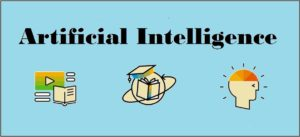• Reasoning
• Learning
• Problem Solving
• Perception
• Linguistic Intelligence

Let us go through all the components briefly −

• Reasoning − It is the set of processes that enables us to provide basis for judgement, making decisions, and prediction. There are broadly two types −
Inductive Reasoning Deductive Reasoning
It conducts specific observations to makes broad general statements. It starts with a general statement and examines the possibilities to reach a specific, logical conclusion.
Even if all of the premises are true in a statement, inductive reasoning allows for the conclusion to be false. If something is true of a class of things in general, it is also true for all members of that class.
Example − “Nita is a teacher. Nita is studious. Therefore, All teachers are studious.” Example − “All women of age above 60 years are grandmothers. Shalini is 65 years. Therefore, Shalini is a grandmother.”
• Learning − It is the activity of gaining knowledge or skill by studying, practising, being taught, or experiencing something. Learning enhances the awareness of the subjects of the study.The ability of learning is possessed by humans, some animals, and AI-enabled systems. Learning is categorized as −
• Auditory Learning − It is learning by listening and hearing. For example, students listening to recorded audio lectures.
• Episodic Learning − To learn by remembering sequences of events that one has witnessed or experienced. This is linear and orderly.
• Motor Learning − It is learning by precise movement of muscles. For example, picking objects, Writing, etc.
• Observational Learning − To learn by watching and imitating others. For example, child tries to learn by mimicking her parent.
• Perceptual Learning − It is learning to recognize stimuli that one has seen before. For example, identifying and classifying objects and situations.
• Relational Learning − It involves learning to differentiate among various stimuli on the basis of relational properties, rather than absolute properties. For Example, Adding ‘little less’ salt at the time of cooking potatoes that came up salty last time, when cooked with adding say a tablespoon of salt.
• Spatial Learning − It is learning through visual stimuli such as images, colors, maps, etc. For Example, A person can create roadmap in mind before actually following the road.
• Stimulus-Response Learning − It is learning to perform a particular behavior when a certain stimulus is present. For example, a dog raises its ear on hearing doorbell.
• Problem Solving − It is the process in which one perceives and tries to arrive at a desired solution from a present situation by taking some path, which is blocked by known or unknown hurdles.Problem solving also includes decision making, which is the process of selecting the best suitable alternative out of multiple alternatives to reach the desired goal are available.
• Perception − It is the process of acquiring, interpreting, selecting, and organizing sensory information.Perception presumes sensing. In humans, perception is aided by sensory organs. In the domain of AI, perception mechanism puts the data acquired by the sensors together in a meaningful manner.
• Linguistic Intelligence − It is one’s ability to use, comprehend, speak, and write the verbal and written language. It is important in interpersonal communication.

### Difference between Human and Machine Intelligence

• Humans perceive by patterns whereas the machines perceive by set of rules and data.
• Humans store and recall information by patterns, machines do it by searching algorithms. For example, the number 40404040 is easy to remember, store, and recall as its pattern is simple.
• Humans can figure out the complete object even if some part of it is missing or distorted; whereas the machines cannot do it correctly.